# MySQL的前缀索引及Oracle的类似实现

+关注继续查看

MySQL有一个很有意思的索引类型，叫做前缀索引，它可以给某个文本字段的前面部分单独做索引，从而降低索引的大小。

MySQL的前缀索引

MySQL的前缀索引指的是对指定的栏位的前面几位建立的索引。

Altertable Table_Name add key(column_name(prefix_len));

Createindex index_name on Table_Name(column_name(prefix_len));

Select ..from table_name where column_name=’…’;

delimiter;;

dropFUNCTION if exists random_str;;

CREATEFUNCTION random_str(n int) RETURNS varchar(30000)

begin

declarereturn_str varchar(30000) default "";

declare iint default 0;

whilelength(return_str) < n do

setreturn_str=concat(return_str,md5(rand()));

endwhile;

returnsubstring(return_str,1,n);

end;;

CREATETABLE TEST_PREFIX_IND (

ID INT(10) PRIMARY KEY AUTO_INCREMENT,

NORMAL_STR VARCHAR(20) ,

LONG_STR VARCHAR(1000),

TEXT_STR TEXT,

BLOB_STR BLOB

);

drop  procedure if exists init_test_prefix_ind;;

createprocedure init_test_prefix_ind(n int)

begin

declare iint default 0;

while i< n do

insertinto test_prefix_ind(NORMAL_STR,long_str,  TEXT_STR,BLOB_STR)

values(random_str(20),random_str(rand()*1000+1),random_str(rand()*1000+1),random_str(rand()*300+1));

seti=i+1;

endwhile;

end;;

callinit_test_prefix_ind(10000);;

altertable test_prefix_ind add key(LONG_STR);;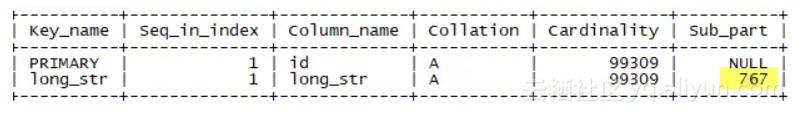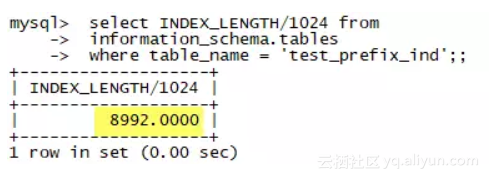mysql> alter table test_prefix_ind add key(text_str);

ERROR 1170 (42000): BLOB/TEXT column 'text_str' used in key specification without a key length

mysql> alter table test_prefix_ind add key(blob_str);;

ERROR 1170 (42000): BLOB/TEXT column 'blob_str' used in key specification without a key length

alter table test_prefix_ind add key(text_str(30));;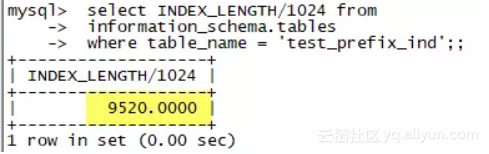alter table test_prefix_ind add key(blob_str(30));;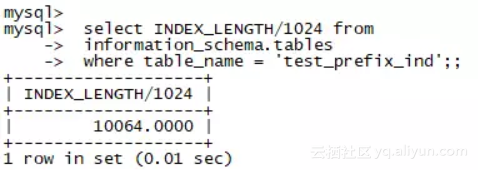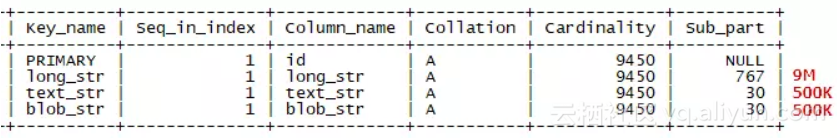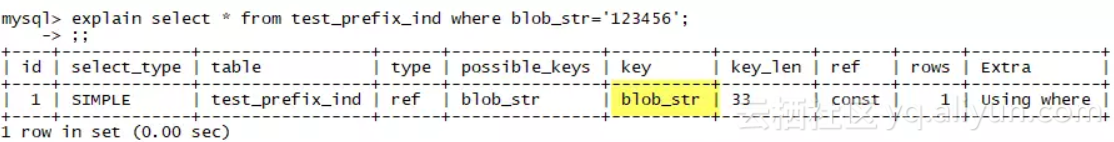Select count(distinct substr(long_str,1,5))/count(*) from test_prefix_ind;

select R,count(distinct substr(long_str,1,R))/count(*)

from

(SELECT @rownum:=ceil(@rownum*1.4) AS  R

FROM (SELECT @rownum:=1) r,test_prefix_ind limit 1,10

) R,test_prefix_ind T

group by R;;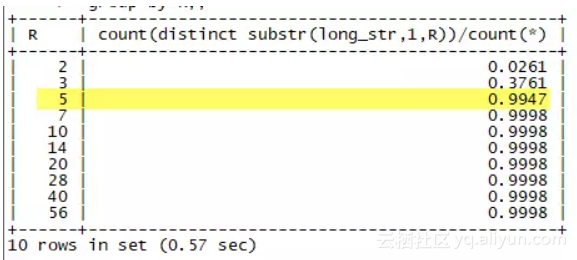alter table test_prefix_ind add key(long_str(5));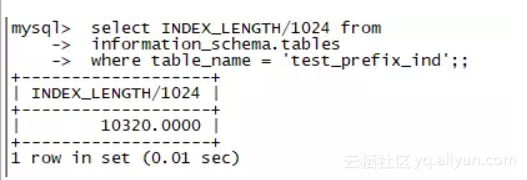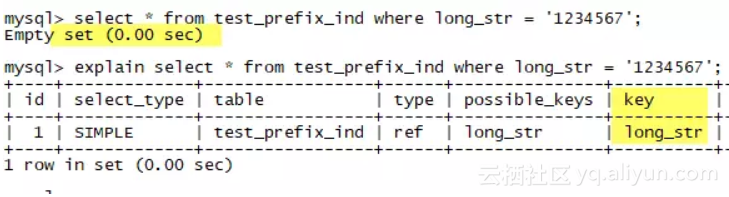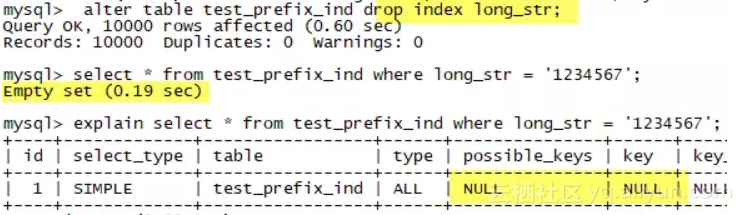Oracle的类似实现

Create index index_name on table_name(substr(column_name,1,<length>) );

Create table test_substr as

select object_id,object_name||dbms_random.string('x',dbms_random.value(1,1000) as object_name,created from all_objects ,

(select * from dual connect by level < 100)

where rownum < 10000;

Create index test_substr_inx on test_substr(substr(object_name,1,5));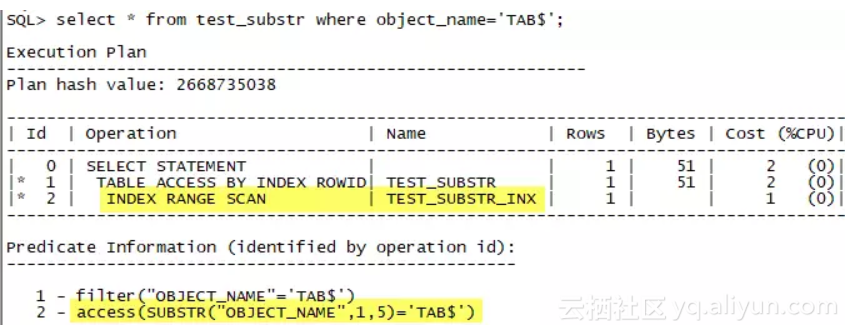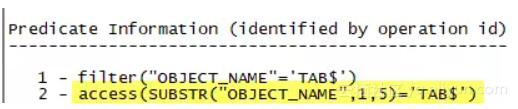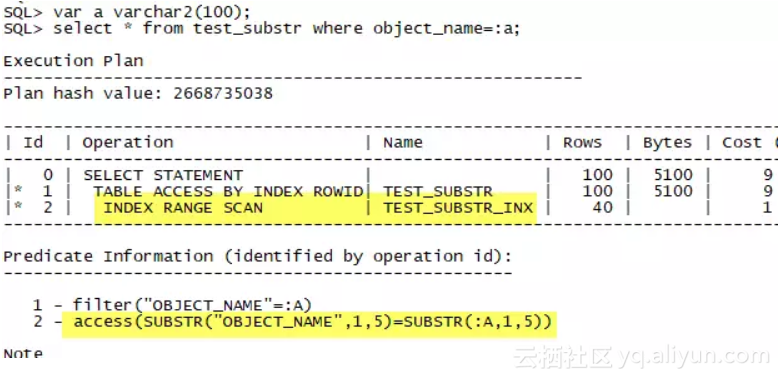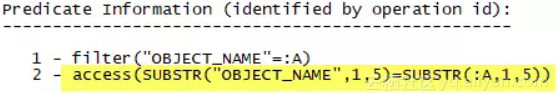select * from test_substr where object_name=:a;

select * from test_substr where object_name=:a and substr(object_name,1,5)=substr(:a,1,5);

SELECT * FROM TEST_SUBSTR WHERE OBJECT_NAME=:A AND SUBSTR(OBJECT_NAME,1,5)=SUBSTR(:A,1,5);

Create index test_substr_inx2 on test_substr(object_name);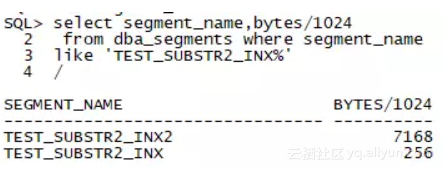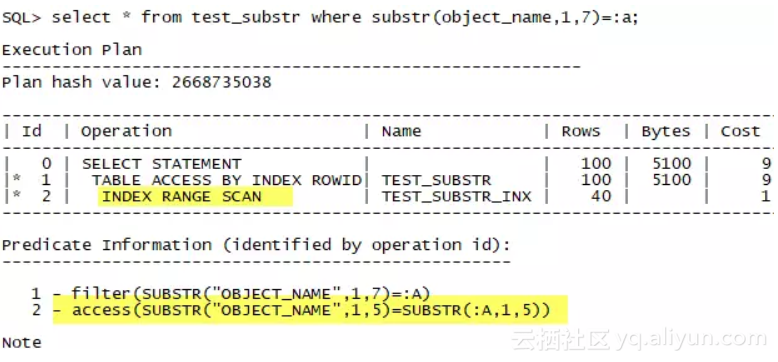select * from test_substr

where substr(object_name,1,<N>)=:a;

select * from test_substr

where substr(object_name,1,<N>)=:a and substr(object_name,1,5)=substr(:a,1,5);

Oracle时间、数字上的前缀索引

Create index test_trunc_date_inx on test_substr(trunc(created));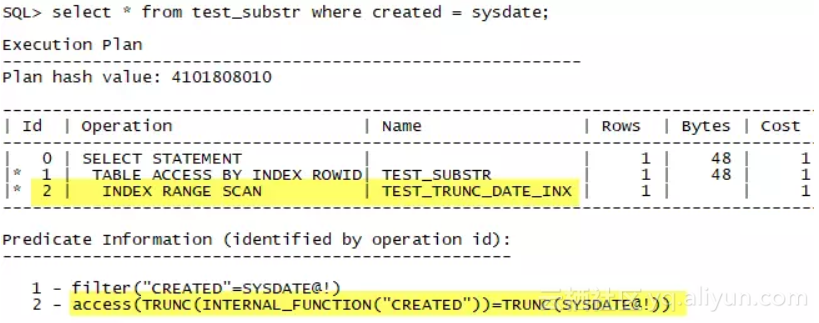create index test_trunc_number on TEST_SUBSTR(trunc(object_id));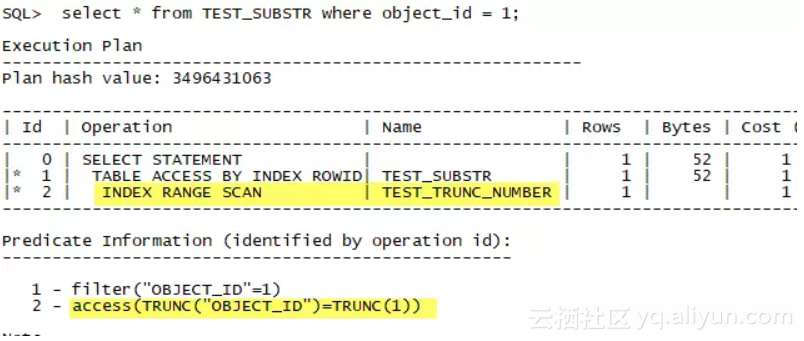create table test_scale (object_name varchar2(5));

insert into test_scale select substr(object_name,1,5) from all_objects;

create index test_scale_str_inx in test_scale(object_name);

select * from  test_scale where object_name = 'DBA_TABLES';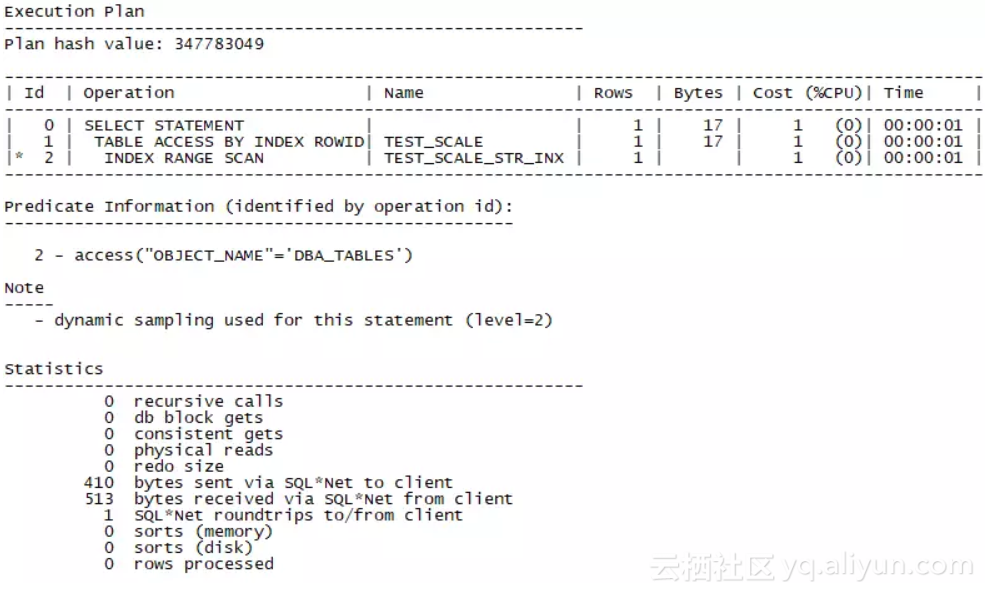|
9月前
|
SQL 关系型数据库 MySQL
MYSQL 批量修改表前缀与删除数据表 sql 语句
MYSQL 批量修改表前缀与删除数据表 sql 语句
145 0
|
9月前
|
SQL 关系型数据库 MySQL
Mysql中索引的最左前缀原则图文剖析（全）

118 0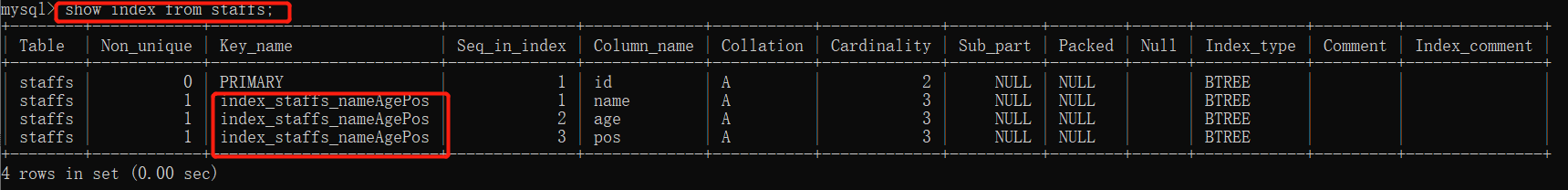|
11月前
|

91 0
|
SQL 存储 关系型数据库
MySQL的最左前缀原则
MySQL的最左前缀原则
741 0
|

MySQL前缀索引上限案例分析

1332 0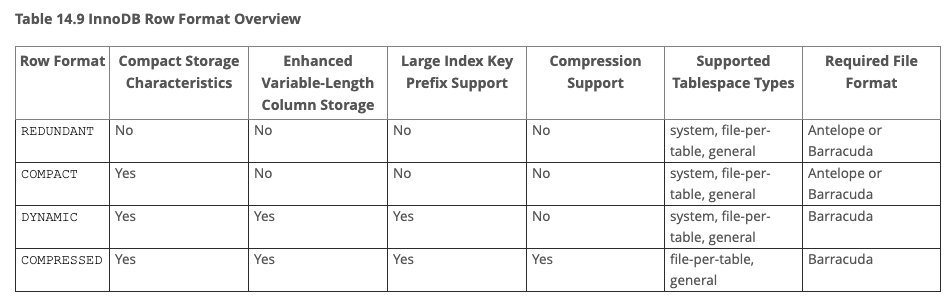|
SQL Oracle 关系型数据库
|
MySQL 关系型数据库 索引